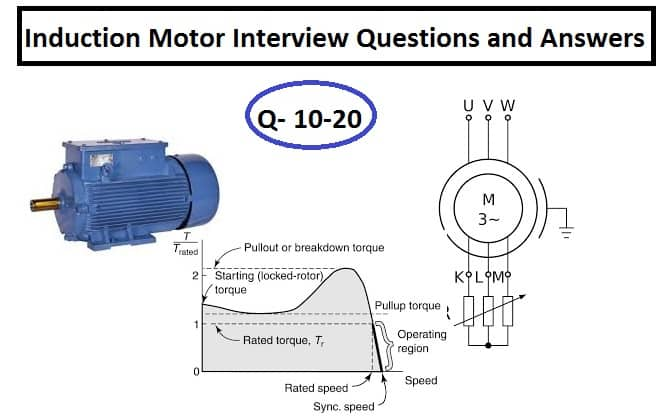# Induction Motor Interview Questions and Answers – Q 10-20Q.11 What are the types of induction motors?

There are two types of induction motor.

• Squirrel Cage Induction Motor – The rotor has short-circuited conductor.
• Slip Ring Induction motor   – Rotor is wound type and rotor is connected to GRR, LRS or VPLRS

Q.12 What are the applications of a slip ring induction motor?

The slip ring induction motor is used for driving the loads which demand higher starting torque. The starting torque of the motor gets increased because of the increased rotor resistance.

The external resistance is added to the rotor conductor and the resistance is reduced as the motor is accelerated. When the motor accelerates up to its base speed the external resistance is short-circuited and the motor is equivalent to a squirrel cage induction motor.

The slip ring induction motor is used for driving the rotary kiln, bucket elevator etc.

Q.13 What are the importance of slip in induction motor?

The induction motor is called an asynchronous motor because the actual speed of the motor is always less than its synchronous speed. The difference in the actual speed and the synchronous speed is called slip. The torque of the motor depends on the slip. The more the slip, the more the torque. The torque of the induction motor is ;

T= slip*Stator voltage*Rotor current* Rotor power factor.

When the load demands more torque, the speed of the motor reduces and the torque increases.If the slip of the motor is zero, no torque will be produced because in this case, the rotor induced voltage and current is zero.

Q.14 What is the formula of the synchronous speed of an induction motor?

The formula of the synchronous speed(Ns) is as given below.

Ns = 120 f/P

Where,

Ns = Synchronous speed in RPM

f = Frequency (Hz)

P = Number of poles

Q.15 Why induction motor draws a large current at the start?

The induction motor draws a large current during acceleration because of the following reasons.

1. The rotor of the induction motor has high inductive impedance because the rotor frequency is equal to the stator supply frequency and the maximum voltage is induced in the rotor as the slip is unity.
2. fr=s*fs and Er=s* Max. Er
3. As the motor accelerates towards its base speed the rotor frequency reduces according to fr-Slip*fs and Er=S*Max. E2 and, the rotor XL reduces accordingly. In a nutshell, the power factor of the rotor is poor.
4. At the start, the magnetizing current and rotor inductive current get added and the large part of the current is lagging behind the applied stator voltage.
5. As the motor attains its full speed the rotor inductive current reduces and the active current increases, the net stator current reduces.

Q.16 What is the difference between slipring (wound-rotor) and squirrel cage motor?

Both types of induction motor function on the principle of Faraday’s law of electromagnetic induction.

The squirrel cage induction motor has a rotor with short circuit end rings. The rotor has a fixed resistance. The squirrel cage induction motor has low starting torque.

In the slip ring induction motor, the rotor is an open circuit and the external resistance is added to the rotor resistance. The rotor resistance gets increased when external resistance is added. Therefore, starting torque of the motor gets improved. The resistance is cut off as the motor accelerates towards its base speed. Once the base speed is obtained, the slip ring is a short circuit and now the motor works like a squirrel cage induction motor.

Q.17 What type of materials are used for slip rings?

The material should have the following characteristics.

• Wear-resistant
• Electrical conductive- Should have minimum resistance

The slip rings are made of steel, copper, or phosphorous bronze. The material of the slip rings must be harder than the material of carbon brushes in order to ensure no wear of the slip rings.

Q.18 What is the purpose of slip rings and brushes?

A slip ring is an electro-mechanical device that allows the transmission of rotor power to a stationary part like GRR or LRS.

A brush is a device that collects current from the slip rings, and then the current flows in the rotor starter circuit. The slip rings and carbon brushes are used in the slip ring motor to connect the rotor to external resistance in order to increase the starting torque.

Q.19 What is the slip of the induction motor?

The rotor speed is always lagging with respect to the synchronous speed of the induction motor. The difference between the speed of the rotor and the speed of the synchronous speed is called slip.

slip(s) = Ns – N

Ns = Synchronous speed of rotating magnetic field

N = Actual speed of the rotor

Q.20  What are the types of induction motors?

On the basis of the supply system

1. Single-phase squirrel cage induction motor ( Not self starting)
2. Three-phase squirrel cage induction motor (self-starting)

On the basis of Construction

1. Three-phase squirrel cage induction motor
2. Three-phase slip ring induction motor

Related Posts